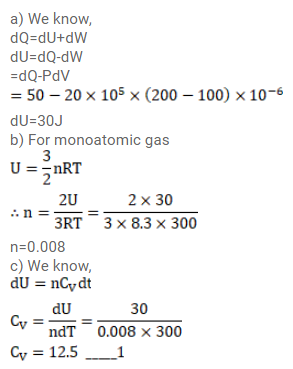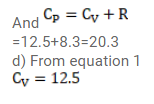# An ideal gas expands fromQuestion:

An ideal gas expands from $100 \mathrm{~cm}^{3}$ to $200 \mathrm{~cm}^{3}$ at a constant pressure of $2.0 \times 10^{5} \mathrm{~Pa}$ when $50 \mathrm{~J}$ of heat is supplied to it. Calculate

(a) the change in internal energy of the gas,

(b) the number of moles in the gas if the initial temperature is 300k,

(c) the molar heat capacity $\mathrm{C}_{\mathrm{p}}$ at constant pressure and

(d) the molar heat capacity $\mathrm{C}_{\mathrm{v}}$ at constant volume.

Solution: